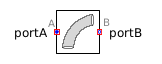1D Lookup Table - MapleSim Help

1D Lookup Table

Generate one or more output signals based on values in a one-dimensional look-up tableDescription The 1D Lookup Table (or Lookup Table 1 D) component generates an output signal by interpolating based on a table and input. The input data values of the table (that is, the first column of values) must be increasing; however, a discontinuous function can be created by repeating an input value.Data Source Options

The data source parameter selects the source for the data. It can be either file, attachment, or inline.

 • file: the data is saved in an Excel or a CSV file on the hard drive. Use the data parameter to browse to and select the data file.
 • attachment: the data set is attached to the MapleSim model. Use the data parameter to select the attachment that contains the data set.
 Note: In the Attachments pane, your data set file must be attached in the Data Sets category. For more information, see Attaching a File to a Model.
 – Attach a Microsoft® Excel® (.xls/.xlsx) or comma separated value (.csv) file containing the data values to the model.
 – Generate a data set in the Apps Manager tab using either the Data Generation app or Random Data Generation app. Data sets that you generate have the .csv file extension. For more information about MapleSim apps, see Opening MapleSim Apps and Templates.
 • inline: enter the data table in the table parameter as an m (rows) by n (columns) matrix.
 – To change the dimensions of table, right-click (Control-click for Mac®) the parameter field and select Edit Matrix Dimensions. In the Matrix Dimensions dialog, enter values for the number or rows and columns and then click OK.

For all data source options, the first column in the data table corresponds to the input value, $u$. The other columns, $2$ through n, represent the output data.

The columns parameter selects which data column to output if your data table has more than two columns. By default, the second column, $\left[2\right]$, is output.

You can output an array by entering an array in columns. For example, set columns to $\left[2,3,4\right]$ to output a three-element array corresponding to the data in the second, third, and fourth columns of your data table.Connections

 Name Description Modelica ID $u$ Real input u $y$ Real output of dimension $n$, the length of the $\mathrm{columns}$ parameter yParametersGeneral Parameters

 Name Default Units Description Modelica ID $\mathrm{data source}$ $\mathrm{inline}$ Data source datasourcemode $\mathrm{MIMO}$ $\mathrm{false}$ True (checked) means the size of the input signal should match the size of the columns parameter MIMO $\mathrm{table}$ $\left[\begin{array}{ccc}0& 1& 1\end{array}\right]$ Data set table $\mathrm{file name}$ $\mathrm{DataFileName}$ Enter the file (complete path) where matrix is stored fileName $\mathrm{data}$ Attached data set data $\mathrm{columns}$ $\left[2\right]$ Data columns used columns $\mathrm{skip rows}$ $0$ Number of header rows to skip skiprows $\mathrm{smoothness}$ Linear interpolation Selects interpolation: linear, cubic spline, or none smoothnessModelica Standard Library The component described in this topic is from the Modelica Standard Library. To view the original documentation, which includes author and copyright information, click here.